# How to use the LOWER function in Excel

The LOWER function in excel is used to change text case to the lower case. In this article, we will explore how you can use LOWER function to do some really important tasks. Let’s get started.

Excel LOWER Function Syntax

=LOWER(text)

Text: it is the text or string that you want to convert in lower-case.

Excel LOWER function Example 1:
In this example, we just want to get lower case of text of input from user. So in column A user will enter their name. It can be in lower, upper, proper or irregular styled text. In Column B, we just will get the lower case text of A column.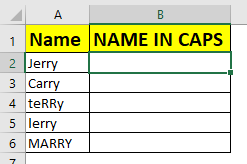Write the below formula in B1 and copy down.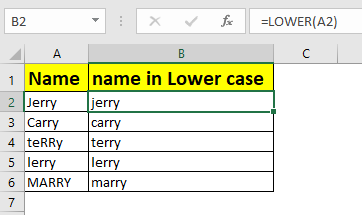Excel LOWER Example 2: Getting Only Lower Case Inputs
Many times you would want the user to input only lower case letters. In this case, we would put a data validation in excel.
Here, we have a column called verification code. Let’s say you have some guest invited in secret party. You have sent a secret code to them. They will enter their secret code in excel column. The trick is, each code should be in lower case. So, if any user puts a non lower case input, it will show error.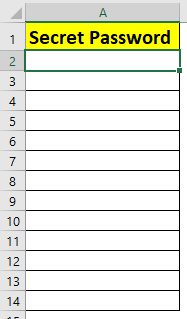1. Select first cell, Here A2.
2. Go to Data tab ? Data validation ?
3. Select Custom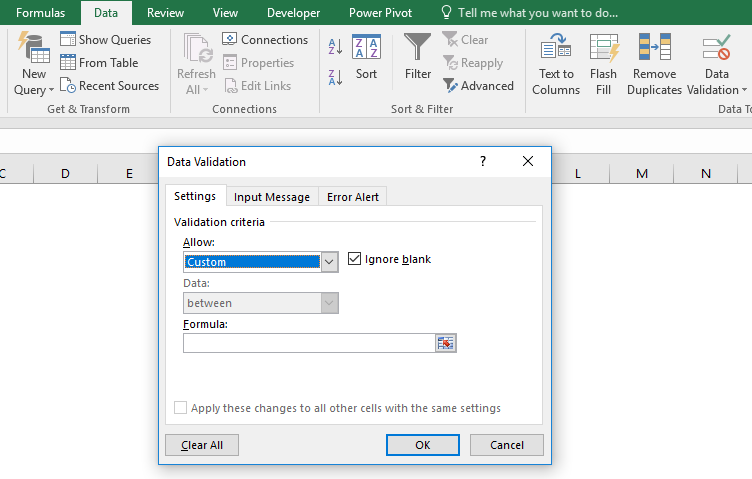1. Write this formula in the formula section. Click OK. Copy this cell down.
=EXACT(A2,LOWER(A2))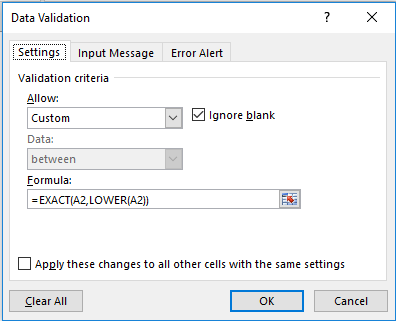Now try to enter any text that is non-lower case input.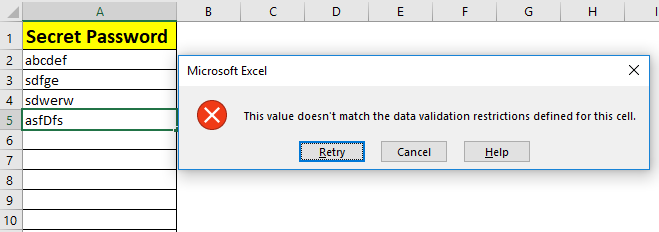It will not except the input.

How it Works
Here, we compare text entered in cell, with LOWER case of that text using EXACT function. If they don’t match, excel shows invalid input prompt.

So yeah guys, this how you use LOWER function in excel. Let me know if you have any doubt about this function of excel in the comments section below.

Related Articles:

How to Use UPPER function in Excel

How to Use PROPER function in Excel

How to use EXACT Function in Excel

How to do Case Sensitive Lookup in Excel

Popular Articles:

50 Excel Shortcuts to Increase Your Productivity

How to use the VLOOKUP Function in Excel

How to use the COUNTIF in Excel 2016

Terms and Conditions of use

The applications/code on this site are distributed as is and without warranties or liability. In no event shall the owner of the copyrights, or the authors of the applications/code be liable for any loss of profit, any problems or any damage resulting from the use or evaluation of the applications/code.